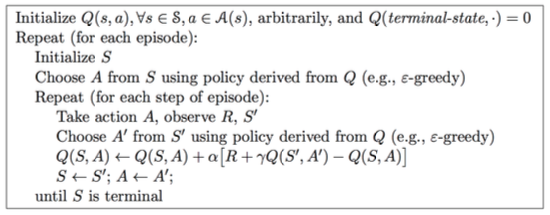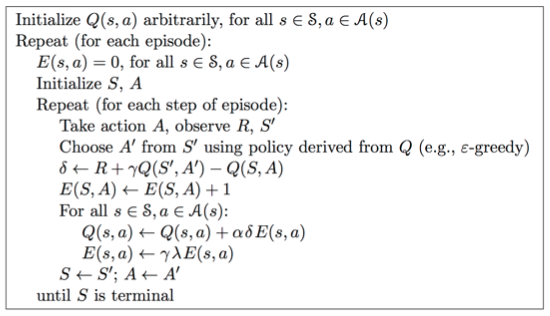# [Reinforcement Learning] Model-Free Control

• MDP model is unknown, but experience can be sampled.
• MDP model is known, but is too big to use, except by samples.

# On-policy Learning vs. Off-policy Learning

On-policy Learning：

• "Learn on the job"
• Learn about policy $\pi$ from experience sampled from $\pi$（即采样的策略与学习的策略一致）

Off-policy Learning：

• "Look over someone's shoulder"
• Learn about policy $\pi$ from experience sampled from $\mu$（即采样的策略与学习的策略不一致）

# On-Policy Monte-Carlo Learning

## Generalized Policy Iteration

![](https://img2018.cnblogs.com/blog/764050/201810/764050-20181030094138877-1709997119.png)

### Model-Free 策略评估

• 基于 $V(s)$ 的贪婪策略提升需要 MDP 已知：

$\pi'(s) = \arg\max_{a\in A}\Bigl(R_{s}^{a}+P_{ss'}^{a}V(s')\Bigr)$

• 基于 $Q(s, a)$ 的贪婪策略提升不需要 MDP 已知，即 Model-Free：

$\pi'(s) = \arg\max_{a\in A}Q(s, a)$

### Model-Free 策略提升

$\pi(a|s) = \begin{cases} &\frac{\epsilon}{m} + 1 - \epsilon, &\text{if } a^*=\arg\max_{a\in A}Q(s, a)\\ &\frac{\epsilon}{m}, &\text{otherwise} \end{cases}$

![](https://img2018.cnblogs.com/blog/764050/201810/764050-20181030094153831-1123656335.png)

## GLIE

![](https://img2018.cnblogs.com/blog/764050/201810/764050-20181030094208835-610523224.png)

$\epsilon_{k} = \frac{1}{k}$

### GLIE Monto-Carlo Control

GLIE Monto-Carlo Control：

• 对于 episode 中的每个状态 $S_{t}$ 和动作 $A_t$

$N(S_t, A_t) ← N(S_t, A_t) + 1 \\ Q(S_t, A_t) ← Q(S_t, A_t) + \frac{1}{N(S_t, A_t)}(G_t - Q(S_t, A_t))$

• 基于新的动作价值函数提升策略：

$\epsilon ← \frac{1}{k}\\ \pi ← \epsilon\text{-greedy}(Q)$

# On-Policy Temporal-Difference Learning

## Sarsa

• 低方差
• Online
• 非完整序列

• 使用 TD 来计算 $Q(S, A)$
• 仍然使用 $\epsilon$-greedy 策略提升
• 每一个 step 进行更新

• 更新动作价值函数
![](https://img2018.cnblogs.com/blog/764050/201810/764050-20181030094221840-942626866.png)
- Control
![](https://img2018.cnblogs.com/blog/764050/201810/764050-20181030094230851-277293106.png)

Sarsa算法的伪代码如下：## Sarsa(λ)

n-step Sarsa returns 可以表示如下：
$n=1$ 时：$q_{t}^{(1)} = R_{t+1} + \gamma Q(S_{t+1})$
$n=2$ 时：$q_{t}^{(2)} = R_{t+1} + \gamma R_{t+2} + \gamma^2 Q(S_{t+2})$
...
$n=\infty$ 时：$q_{t}^{\infty} = R_{t+1} + \gamma R_{t+2} + ... + \gamma^{T-1} R_T$

n-step Sarsa 更新公式：

$Q(S_t, A_t) ← Q(S_t, A_t) + \alpha (q_t^{(n)} - Q(S_t, A_t))$![](https://img2018.cnblogs.com/blog/764050/201810/764050-20181030094258849-595223970.png)

# Off-Policy Learning

Off-Policy Learning 的特点是评估目标策略 $\pi(a|s)$ 来计算 $v_{\pi}(s)$ 或者 $q_{\pi}(s, a)$，但是跟随行为策略 $\{S_1, A_1, R_2, ..., S_T\}\sim\mu(a|s)$

Off-Policy Learning 有什么意义？

• Learn from observing humans or other agents
• Re-use experience generated from old policies $\pi_1, \pi_2, ..., \pi_{t-1}$
• Learn about optimal policy while following exploratory policy
• Learn about multiple policies while following one policy

## 重要性采样

\begin{align} E_{X\sim P}[f(X)] &= \sum P(X)f(X)\\ &= \sum Q(X)\frac{P(X)}{Q(X)}f(X)\\ &= E_{X\sim Q}[\frac{P(X)}{Q(X)}f(X)] \end{align}

### Off-Policy MC 重要性采样

$G_t^{\pi/\mu} = \frac{\pi(A_t|S_t)}{\mu(A_t|S_t)} \frac{\pi(A_{t+1}|S_{t+1})}{\mu(A_{t+1}|S_{t+1})}...\frac{\pi(A_T|S_T)}{\mu(A_T|S_T)}G_t$

$V(S_t) ← V(S_t) + \alpha\Bigl(\color{Red}{G_t^{\pi/\mu}}-V(S_t)\Bigr)$

• Cannot use if $\mu$ is zero when $\pi$ is non-zero
• 重要性采样会显著性地提升方差

### Off-Policy TD 重要性采样

TD 是单步的，所以使用策略 $\pi$ 产生的 TD targets 来评估 $\mu$

$V(S_t) ← V(S_t) + \alpha\Bigl(\frac{\pi(A_t|S_t)}{\mu(A_t|S_t)}(R_{t+1}+\gamma V(S_{t+1}))-V(S_t)\Bigr)$

• 方差比MC版本的重要性采样低很多

## Q-Learning

• 不需要重要性采样
• 使用行为策略选出下一步的动作：$A_{t+1}\sim\mu(·|S_t)$
• 但是仍需要考虑另一个后继动作：$A'\sim\pi(·|S_t)$
• 朝着另一个后继动作的价值更新 $Q(S_t, A_t)$

$Q(S_t, A_t) ← Q(S_t, A_t) + \alpha\Bigl(R_{t+1}+\gamma Q(S_{t+1}, A')-Q(S_t, A_t)\Bigr)$

• 行为策略和目标策略均改进
• 目标策略 $\pi$ 以greedy方式改进：

$\pi(S_t) = \arg\max_{a'}Q(S_{t+1}, a')$

• 行为策略 $\mu$$\epsilon$-greedy 方式改进
• Q-Learning target：

\begin{align} &R_{t+1}+\gamma Q(S_{t+1}, A')\\ =&R_{t+1}+\gamma Q\Bigl(S_{t+1}, \arg\max_{a'}Q(S_{t+1}, a')\Bigr)\\ =&R_{t+1}+\max_{a'}\gamma Q(S_{t+1}, a') \end{align}

Q-Learning 的 backup tree 如下所示：

![](https://img2018.cnblogs.com/blog/764050/201810/764050-20181030094313903-820222072.png)

Q-learning control converges to the optimal action-value function, $Q(s, a)→q_*(s, a)$

Q-Learning 算法具体的伪代码如下：

![](https://img2018.cnblogs.com/blog/764050/201810/764050-20181030094323853-141348712.png)

• TD target 公式不同
• Q-Learning 中下一步的动作从行为策略中选出，而不是目标策略

# DP vs. TD

![](https://img2018.cnblogs.com/blog/764050/201810/764050-20181030094333833-1951913901.png) ![](https://img2018.cnblogs.com/blog/764050/201810/764050-20181030094341855-1641614291.png)

# Reference

posted @ 2018-10-31 10:40  Poll的笔记  阅读(...)  评论(...编辑  收藏